###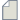Author Topic: Thermoelectric coefficients vs Temperature  (Read 202 times)

0 Members and 1 Guest are viewing this topic.

####wisam

• New ATK user
•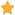• Posts: 4
• Country: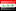• Reputation: 0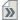##### Thermoelectric coefficients vs Temperature
« on: May 3, 2023, 23:49 »
Hello.

I am having a problem with obtaining the Thermoelectric coefficients vs Temperature.
I have followed this tutorial and got the thermal conductance of phonons vs Temperature graph, and I got it one.
https://docs.quantumatk.com/tutorials/thermoelectrics_cnt_isotope/thermoelectrics_cnt_isotope.html
But the graphs I want are the thermal conductance of electrons, Seebeck coefficient, Peltier coefficients, and ZT vs Temperature. Is there any way I can get ZT vs Temperature with Quantum ATK?

Thank you.

####Anders Blom

• QuantumATK Staff
• Supreme ATK Wizard
•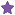• Posts: 5221
• Country:• Reputation: 87##### Re: Thermoelectric coefficients vs Temperature
« Reply #1 on: May 4, 2023, 08:50 »
This can be done with some easy Python scripting. If you have an HDF5 file with a transmission spectrum and phonon transmission spectrum, the following code will compute these quantities for a given temperature. Then you just have to loop over the temperature and plot it.
Code: python
``````transmission_spectrum = nlread("file.hdf5", TransmissionSpectrum)[-1]
temperature = 300*Kelvin

k0, k1, k2 = evaluateMoments(transmission_spectrum, temperature)

thermal_conductance_phonons = phonon_transmission_spectrum.thermalConductance(temperature)

conductance =  k0
peltier_coefficient = -k1/(k0*Units.e)
seebeck_coefficient = -k1/(k0*Units.e*temperature)
thermal_conductance_electrons=  (k2*k0-k1*k1)/(temperature*k0*Units.e**2)
total_thermal_conductance = thermal_conductance_electrons + thermal_conductance_phonons
zt = conductance*seebeck_coefficient**2*temperature/total_thermal_conductance
``````
Sorry if I made any typos, I had no good case to check against right at hand, but hopefully you get the idea. If you have a spin-polarized system, each quantity k0, k1, k2 needs to be separately evaluated for up and down spin and then all quantities are added up (a bit complex, we can add this later in this discussion if relevant).

####wisam

• New ATK user
•• Posts: 4
• Country:• Reputation: 0##### Re: Thermoelectric coefficients vs Temperature
« Reply #2 on: May 5, 2023, 01:02 »
This can be done with some easy Python scripting. If you have an HDF5 file with a transmission spectrum and phonon transmission spectrum, the following code will compute these quantities for a given temperature. Then you just have to loop over the temperature and plot it.
Code: python
``````transmission_spectrum = nlread("file.hdf5", TransmissionSpectrum)[-1]
temperature = 300*Kelvin

k0, k1, k2 = evaluateMoments(transmission_spectrum, temperature)

thermal_conductance_phonons = phonon_transmission_spectrum.thermalConductance(temperature)

conductance =  k0
peltier_coefficient = -k1/(k0*Units.e)
seebeck_coefficient = -k1/(k0*Units.e*temperature)
thermal_conductance_electrons=  (k2*k0-k1*k1)/(temperature*k0*Units.e**2)
total_thermal_conductance = thermal_conductance_electrons + thermal_conductance_phonons
zt = conductance*seebeck_coefficient**2*temperature/total_thermal_conductance
``````
Sorry if I made any typos, I had no good case to check against right at hand, but hopefully you get the idea. If you have a spin-polarized system, each quantity k0, k1, k2 needs to be separately evaluated for up and down spin and then all quantities are added up (a bit complex, we can add this later in this discussion if relevant).
Dear Anders Blom I tried hard using Python scripting, but unfortunately, I didn't get a result because I didn't know how to write codes using Python. I want to attach an HDF5 file with the transmission and phonon spectrum, but the site does not allow it because it is too large. Please send me your email for sending the file, and I ask you to help me write the complete code to calculate these quantities with temperature. I desperately need your help. Sincere regards Emal: wisam122@ahoo.com

####Anders Blom

• QuantumATK Staff
• Supreme ATK Wizard
•• Posts: 5221
• Country:• Reputation: 87##### Re: Thermoelectric coefficients vs Temperature
« Reply #3 on: May 5, 2023, 21:56 »
This very simple script prints out the Seebeck and ZT as a function of temperature
Code: python
``````from AddOns.TransportCoefficients.Utilities import evaluateMoments

print(f"T\tSeebeck coefficient (k/e)\tZT")
for T in numpy.arange(100,400,25):

temperature = T*Kelvin

k0, k1, k2 = evaluateMoments(transmission_spectrum, temperature)
conductance = -k0
peltier_coefficient = -k1/(k0*Units.e)
seebeck_coefficient = -k1/(k0*Units.e*temperature)
thermal_conductance_electrons= -(k2*k0-k1*k1)/(temperature*k0*Units.e**2)
thermal_conductance_phonons = phonon_transmission_spectrum.thermalConductance(temperature)
total_thermal_conductance = thermal_conductance_electrons + thermal_conductance_phonons
zt = conductance*seebeck_coefficient**2*temperature/total_thermal_conductance

print(f"{T}\t{seebeck_coefficient.inUnitsOf(Units.boltzmann_constant/Units.e)}\t{zt}")
``````
« Last Edit: May 5, 2023, 21:58 by Anders Blom »